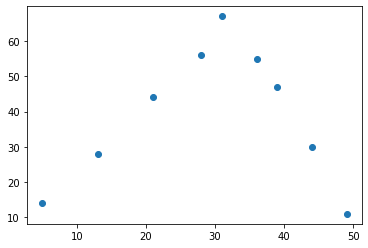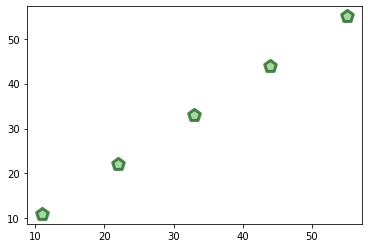# .scatter()

The `.scatter()` method in the matplotlib library is used to draw a scatter plot, showing a relationship between variables.

## Syntax

``````matplotlib.pyplot.scatter(x, y, s, c, marker, cmap, norm, vmin, vmax, alpha, linewidths, edgecolors, plotnonfinite)
``````

Both ‘x’ and ‘y’ parameters are required, and represent float or array-like objects. Other parameters are optional and modify plot features like marker size and/or color.

`.scatter()` takes the following arguments:

• `x` and `y`: Positional arguments of type float or array.
• `s`: A float or an array (of size equal to x or y) specifying marker size.
• `c`: An array or list specifying marker color.
• `marker`: Sets the marker style, specified with a shorthand code (e.g. “.”: point, “o”: circle) or an instance of the class.
• `cmap`: A Colormap instance used to map scalar data to colors. (Default: “viridis”)
• `norm`: Normalization method used to scale scalar data to a range of (0 to 1) before mapping. Linear scaling is default.
• `vmin` and `vmax`: Sets the data range for the colormap (if norm is not specified).
• `alpha`: Sets the transparency value of the markers - range between 0 (transparent) and 1 (opaque).
• `linewidths`: Sets the linewidth of the marker edge.
• `edgecolors`: Sets the edge color of the marker.
• `plotnonfinite`: Boolean value determining whether to plot nonfinite (`inf`, `-inf`, `nan`) values. Default is `False`.

## Examples

Examples below demonstrate the use of `.scatter()` to plot values and vary marker properties.

```import matplotlib.pyplot as plt
x1 = [5, 13, 21, 28, 31, 34, 39, 44, 49]y1 = [14, 28, 44, 56, 67, 53, 47, 30, 11]
plt.scatter(x1, y1)plt.show()
```

Output:```import matplotlib.pyplot as plt
x2 = [11, 22, 33, 44, 55]y2 = [11, 22, 33, 44, 55]
plt.scatter(x2, y2, s=150, c='#88c988', linewidth=3, marker='p' , edgecolor='#175E17', alpha=0.75)plt.show()
```

Output: